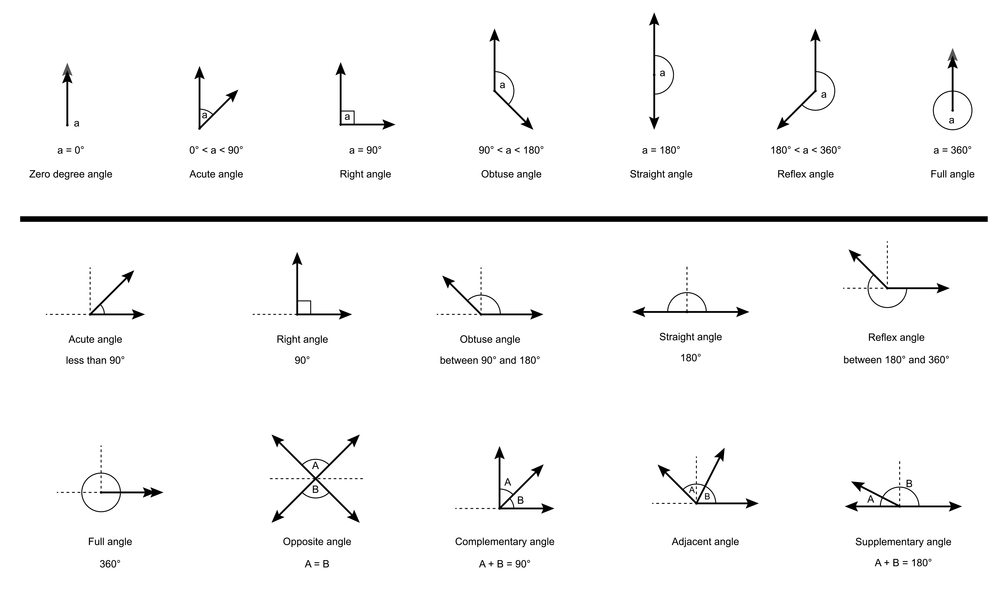# Types of Angles: Acute, Right, Obtuse, Straight, and Reflex## Angle:

In the geometry, the two hands represent two rays meeting at a point and when the two rays have a common endpoint they form an angle. The two rays forming an angle are called the arms of the angle or the segments of the angle and the common endpoint is called the vertex of the angle. The angles are measured in degrees usually. To measure the angles, we usually use an instrument called a protractor, it is in the shape of a semicircle, and the midpoint of the protractor is called the central point from where we measure the angle. The angle at the centre is divided into 180 equal divisions. It goes up to 360 equal divisions if we do a complete turnaround and each of these parts is called degree. The degree is denoted by °. Degrees indicate how open each angle is and on their openings, we classify those angles.  An angle is denoted by the symbol ∠.  There are different types of angles depending on the position of the arms.

## Types of Angles:

The different types of angles are:

1. Acute Angle
2. Right Angle
3. Obtuse Angle
4. Straight Angle
5. Reflex Angle
6. Full Rotation

• Acute Angle:
An angle whose measure is more than zero degree 0° and less than ninety degrees 90° is known as an acute angle. Acute angles are smaller than other angles. If we have a triangle ABC with an angle of 50° at B then one can say that ABC is an acute angle triangle. ∠B is an acute angle since the intersection of AB and CB at B made an angle smaller than 90°.

• Right Angle:
An angle whose measure is ninety degrees (90°) is known as a right angle and it is larger than an acute angle. When the arms of the angle are perpendicular to each other they form a right angle. However, if the angle is more than ninety degrees (90°) then it would not be the right angle. If we have a triangle PQR, PQ and QR are perpendicular to each other or intersect each other at Q forming an angle of ∠Q = 90° making it a right angle.

• Obtuse Angle:
An angle whose measure is more than ninety degrees (90°) and less than one hundred and eighty degrees (180°) is called the obtuse angle. The obtuse angle is bigger than the right angle and the acute angle. An obtuse angle measures between ninety degrees (90°) to one hundred and eighty degrees (180°). One of the ways to calculate Obtuse Angle is if we know the value of the acute angles. For Instance,

Obtuse Angle = 180° – Value of Acute Angle

• Straight Angle:
The angle if the arms of the angle are in an opposite direction to each other is known as the straight angle. In simple words, the type of angle that measures 180 degrees (180°) is called a straight angle. If the angle measure is more than 180° or less than 180° then it cannot be a straight angle. Moreover, it makes a full semi-circle.

• Reflex Angle
An angle whose measure is more than one hundred and eighty degrees (180°) and less than three hundred and sixty degrees (360°) is called the reflex angle. Reflex angle is bigger than the straight angle however, smaller than the full rotation as the reflex  angle is not equal to three hundred and sixty degrees (360°). A reflex angle measures between one hundred and eighty degrees (180°) to three hundred and sixty degrees (360°). One of the ways to calculate Obtuse Angle is if we know the value of the acute angle or the obtuse angle . For Instance,

Reflex Angle = 360° – Value of Acute Angle or Obtuse Angle

• Full Rotation:
If both the arms of the angle overlap each other then they form an angle that measures three hundred and sixty degrees is known as full rotation. In simple words, the type of angle that measures or equals to three hundred and sixty degrees (360°) is known as the full rotation. It is also known as the complete angle due to the fact that it makes a complete circle.

Moreover, Angles can be divided into two types based on their rotation that are positive angles and negative angles.

Positive Angle:

Positive angle is an angle which is in a counter – clockwise direction from base and usually they are used to represent angles in mathematics, geometry etc.

Negative Angle:

Negative angle is an angle which is in a clockwise direction from base and usually they are used to represent negative angles in mathematics, geometry etc.
To make it easier for you, we are going to make a table which you can use for remembering these angles!

 TYPES OF ANGLES ACUTE ANGLE More than 0°Less than 90° RIGHT ANGLE Equals to 90° OBTUSE ANGLE More than 90°Less than 180° STRAIGHT ANGLE Equals to 180° REFLEX ANGLE More than 180°Less than 360° FULL ROTATION Equals to 360°

### Find Top Tutors in Your AreaWith over 3 years of experience in teaching, Chloe is very deeply connected with the topics that talk about the educational and general aspects of a student's life. Her writing has been very helpful for students to gain a better understanding of their academics and personal well-being. I’m also open to any suggestions that you might have! Please reach out to me at chloedaniel402 [at] gmail.com